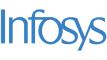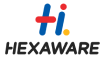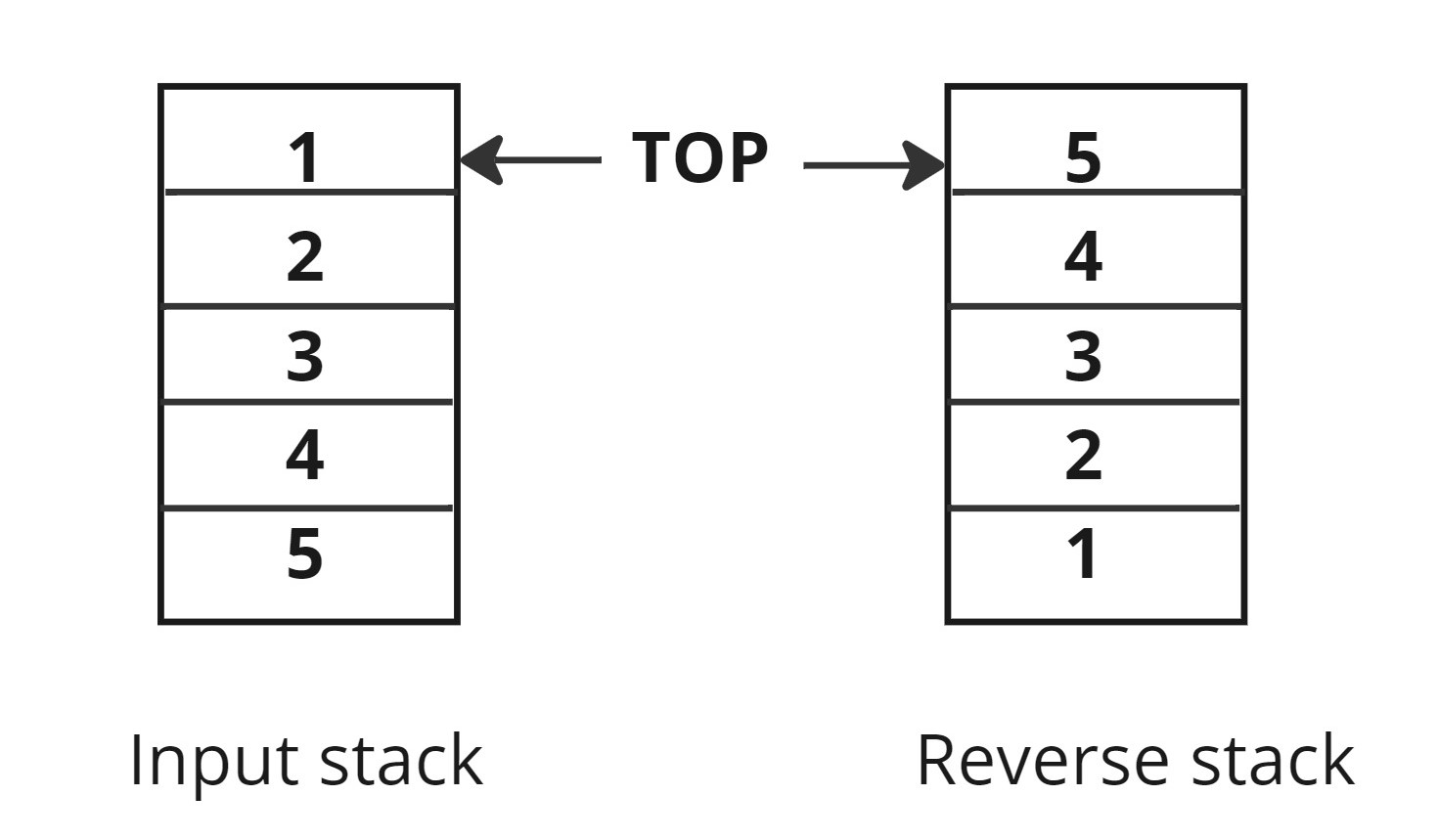New update is available. Click here to update.

# Reverse Stack Using Recursion

Easy0/40
21 mins
80 %230 upvotes+34 more

## Problem Statement

#### Note: You are not allowed to use any extra space other than the internal stack space used due to recursion.

##### Example:
``````Input: [1,2,3,4,5]
Output: [5,4,3,2,1]
``````Detailed explanation ( Input/output format, Notes, Images )
##### Sample Input 1 :
``````3
2 1 3
``````
##### Sample Output 1 :
``````3 1 2
``````
##### Explanation to Sample Input 1 :
``````Reverse of a give stack is 3 1 2 where first element becomes last and last becomes first.
``````
##### Sample Input 2 :
``````2
3 2
``````
##### Sample Output 2 :
``````2 3
``````
##### Constraints :
``````0 <= N <= 10^3
Where 'N' is the number of elements in the given stack.

Time Limit: 1 sec
``````AutocompleteConsole